Function Repository Resource:

# MatrixGeometricMean

Compute the geometric mean of two matrices

Contributed by: Jan Mangaldan
 ResourceFunction["MatrixGeometricMean"][a,b] gives the geometric mean of the matrices a and b.

## Details

The geometric mean of two matrices a and b is the unique positive definite matrix solution x of the equation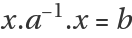, and is the generalization of the usual geometric mean for scalars.
The matrices a and b can be numerical or symbolic, but must be Hermitian and positive definite.
The geometric mean of two matrices can have complex-valued elements.

## Examples

### Basic Examples (2)

The geometric mean of two exact 2×2 symmetric positive definite matrices:

 In:=Out=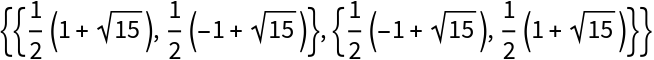The geometric mean is also symmetric and positive definite:

 In:=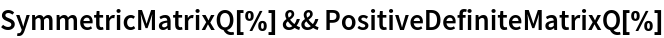Out=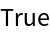### Scope (2)

Two symmetric positive definite matrices:

 In:=Compute the geometric mean with machine arithmetic:

 In:=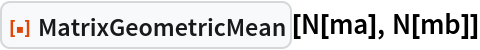Out=Compute the geometric mean with 24-digit precision arithmetic:

 In:=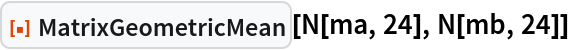Out=Compute the geometric mean of two random Hermitian positive definite matrices:

 In:=Out=### Properties and Relations (6)

MatrixGeometricMean of two 1×1 matrices is equivalent to GeometricMean:

 In:=Out=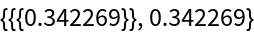The geometric mean is symmetric in its arguments:

 In:=Out=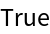The geometric mean of a matrix and the identity is equivalent to the square root of the matrix:

 In:=Out=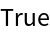If two matrices commute, their geometric mean is equivalent to the square root of their product:

 In:=Out=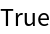In:=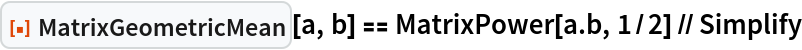Out=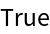The geometric mean can be expressed in terms of MatrixPower:

 In:=Out=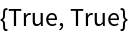The geometric mean can be expressed as an integral:

 In:=Out=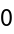## Version History

• 1.0.0 – 06 May 2022